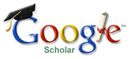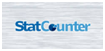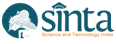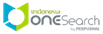### PENGARUH STRES KERJA DAN MOTIVASI KERJA TERHADAP KINERJA KARYAWAN PADA PT. TIRTA MAHAKAM RESOURCES TBK SAMARINDA

Novi Susanti, Mardiana

#### Abstract

The study aims to determine the effect of work stress and work motivation on employee performance. Specifically this study aims to find out how the influence of job stress on employee performance and know how well the influence of work motivation on employee performance, and to know the influence of work stress and work motivation on employee performance at PT. Tirta Mahakam Resources Tbk Samarinda.

The method used is quantitative analysis that is by multiple linear regression analysis. The population in this study is the number of permanent employees in the production of PT. Tirta Mahakam Resources Tbk Samarinda in 2017 as many as 792 population. The sample in this research using simple random sampling by using slovin formula technique, so sample taken as many as 266 samples of employees remain part production PT. Tirta Mahakam Resources Tbk Samarinda.

The results showed that the value of F arithmetic = 143,844, using the significance limit of 0.05, then obtained the significance value is smaller than 0.05 is 0.000, it means that simultaneously the variable work stress and work motivation significantly influence employee performance. The coefficient of determination (R2) is 0,522 or 52,2%, hence coefficient simultaneously in explaining variation or change of dependent variable (Y) found the influence of free variable 52,2%, while the rest is 47,8%. The result of hypothesis testing (H1) through the calculation results obtained t count value of 15,444 with the significance level of 0.000 results is smaller than 0.05, which means that the hypothesis in this study Ho rejected and Ha accepted. This test statistically proves that work stress has positive and dominant effect to employee performance. The result of hypothesis testing (H2) through the calculation results obtained t count value of 3.215 with the significance of the result of 0.001 is smaller than 0.05, which means that the hypothesis in this study Ho rejected and Ha accepted. This test statistically proves that the motivation has a positive effect on the performance of employees of PT. Tirta Mahakam Resources Tbk Samarinda.

#### Keywords

Job Stress, Work Motivation and Employee Performance

#### Full Text:

DOC PDF

DOI: https://doi.org/10.31293/rjabm.v2i2.3734

### Refbacks

• There are currently no refbacks.